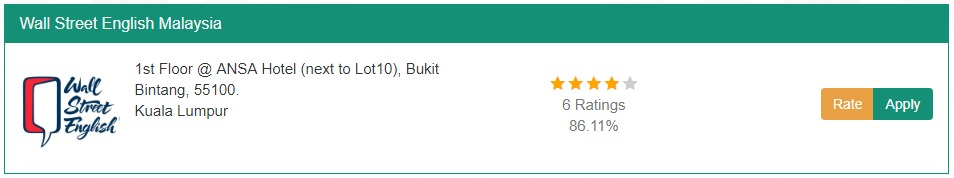## Find Ratings:

At RateMyESL, we strive to enable students, parents, agencies and education professionals to have a transparent outlook to the best ESL centres globally. Most of our staff were ESL students at one point, hence, we understand how important it is to pick the right ESL school, we also understand how important is the opinion of other students who went to an ESL Centre you might be interested to join.

Ratings

How ratings are calculated?

1. We calculate the average of all ratings of each institute. The result of calculating the average would be from 0.0 to 5.0.
2. To get the percentage, we convert the result from 5 to 100 scale.
3. The 5-star rating of each institute is shown after rounding the result of 5 scale.

Example:

6 people have rated an ESL center (Wall Street English Malaysia), the following are all those 6 people's ratings:

4, 3, 3, 4, 5, 5, 4, 4, 5, 4, 5, 4, 5, 5, 5, 5, 5, 5, 5, 5, 5, 5, 5, 5, 4, 4, 4, 4, 3, 3, 5, 5, 3, 3, 4, 3*

###### * note those ratings include all 6 rating questions (Programs, City ..)

Mathematically speaking, we calculate the average of all those ratings as follows:

1- We count the the ratings (Answer is 36)

2- We sum the ratings (Answer is 155)

3- The average would be: sum ÷ count

155 ÷ 36 = 4.3055555555556

Now we have the overall rating of  (Wall Street English Malaysia) which is 4.30/5 and only 4 stars are shown. Then we get the percentage by this formula:

(4.3055555555556 / 5) × 100 = 86.1111111111

Finally, we round up to the second decimal place and the result is 86.11%.Why are we show ratings based on Stars & Percentage at the same?

To show a quantifiable numerical value which can clearly distinguish between ESL centers.